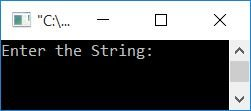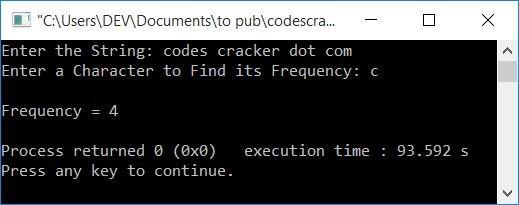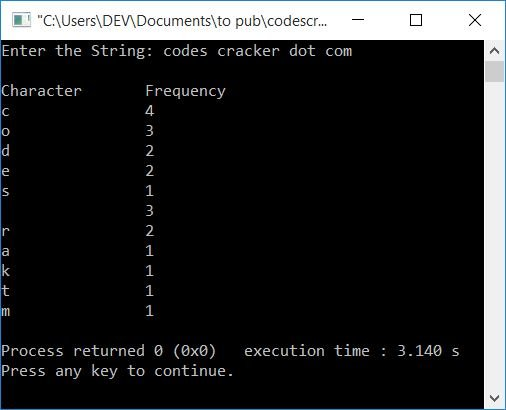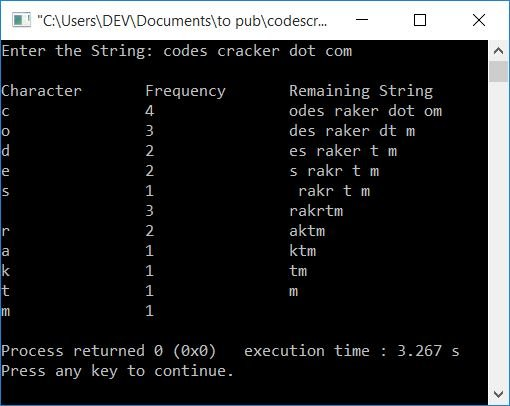# C++ program to find the frequency of characters in a string

In this article, you will learn and get code to find and print the frequency of characters (single and all) in a string using the C++ language. Here is the list of programs available in this article:

The user must enter both characters and strings at run-time.

Note: The frequency of a character in a string is the total number of times the character occurs in the given string. or the occurrence of a character in a string.

## Find the Frequency of a Character in a String

To find the frequency of a character in a string using C++ programming, you have to ask the user to enter the string first, and then ask them to enter the character to find its frequency, as shown in the program given below:

The question is: write a program in C++ to find the frequency of a character in a string. Here is its answer:

```#include<iostream>
#include<stdio.h>
using namespace std;
int main()
{
char str, ch;
int i=0, freq=0;
cout<<"Enter the String: ";
gets(str);
cout<<"Enter a Character to Find its Frequency: ";
cin>>ch;
while(str[i])
{
if(ch==str[i])
freq++;
i++;
}
cout<<"\nFrequency = "<<freq;
cout<<endl;
return 0;
}```

This program was built and runs under the Code::Blocks IDE. Here is its sample run:Now supply any string, say codes cracker dot com, and then a character, say c, to find its frequency after pressing the ENTER key. Here is the sample output:The dry run of the above program with the same user input (as provided in the sample run given above) goes like this:

• Initial values, i=0 and freq=0
• When the user enters the string, then it gets stored in str[] in this way:
• str=c
• str=o
• str=d
• str=e
• str=s
• str=' '
A space
• str=c
• str=r
• and so on up until
• str=m
• And when the user enters a character, say c, then it gets stored in ch.
• Now the execution of the while loop gets started. Its execution will continue until its condition (str[i]) is determined to be false. When the value of i becomes 21, then, because at the 21th index, there is no character left, its condition evaluates to be false.
• The condition str[i] or str (on putting the value of i) or c evaluates to true on the first run of the while loop, so program flow goes inside the loop.
• And the condition of if, that is, ch==str[i] or c==str or c==c, evaluates to be true, therefore program flow goes inside the if's body and the value of freq gets incremented. So freq=1
• Now the value of i also gets incremented, and the program flow goes back and evaluates the condition of the while loop again with the new value of i (1).
• That is, the condition str[i] or str or o evaluates to true again, and the program flow returns to the loop.
• But this time, the condition of if, that is, ch==str[i] or c==str or c==o, evaluates to be false, therefore the value of freq does not gets incremented. So only the value of i gets incremented. Now i=2, and the program flow goes back and evaluates the condition of the while loop again.
• This process continues until its condition is evaluated as false.
• Every time we enter its body, we compare the value of ch with each and every character available in the string str. If a character gets matched, then the value of freq gets incremented.
• Therefore, after exiting the loop, we'll have a variable freq that holds the character frequency. So just print its value as output.

## Determine the Frequency of All Characters in a String

This program asks the user to enter the string alone. Because this program finds and prints the frequency of all characters in a given string.

```#include<iostream>
#include<stdio.h>
#include<string.h>
using namespace std;
int main()
{
char str, ch;
int i, j, k, len, freq=0;
cout<<"Enter the String: ";
gets(str);
len = strlen(str);
cout<<"\nCharacter\tFrequency";
for(i=0; i<len; i++)
{
ch = str[i];
for(j=0; j<len; j++)
{
if(ch==str[j])
{
freq++;
for(k=j; k<(len-1); k++)
str[k] = str[k+1];
len--;
str[len] = '\0';
j--;
}
}
cout<<endl<<ch<<"\t\t"<<freq;
freq=0;
i--;
}
cout<<endl;
return 0;
}```

Here is its sample run with user input, codes cracker dot com:In the preceding program, whenever a character matches a character at any index, we shift all the characters from that index back to one index.

Note: Don't forgot to initialize a null-terminated character (\0) at the last index of the new string.

### Count each character and print the remaining string every time

This program is identical to the previous one. The only difference is that this program also prints the remaining string in the third column:

```#include<iostream>
#include<stdio.h>
#include<string.h>
using namespace std;
int main()
{
char str, ch;
int i, j, k, len, freq=0;
cout<<"Enter the String: ";
gets(str);
len = strlen(str);
cout<<"\nCharacter\tFrequency\tRemaining String";
for(i=0; i<len; i++)
{
ch = str[i];
for(j=0; j<len; j++)
{
if(ch==str[j])
{
freq++;
for(k=j; k<(len-1); k++)
str[k] = str[k+1];
len--;
str[len] = '\0';
j--;
}
}
cout<<endl<<ch<<"\t\t"<<freq<<"\t\t"<<str;
freq=0;
i--;
}
cout<<endl;
return 0;
}```

Here is its sample run with the same user input as provided in the previous program:#### The same program in different languages

C++ Quiz

« Previous Program Next Program »